# Chapter 5 Chemical Reactions Thermodynamics Kinetics Chemical Reactions

• Slides: 35Chapter 5 Chemical Reactions • • • Thermodynamics Kinetics Chemical Reactions Oxidation and Reduction Organic Reactions: Condensation and Hydrolysis Organic Addition Reactions to Alkenes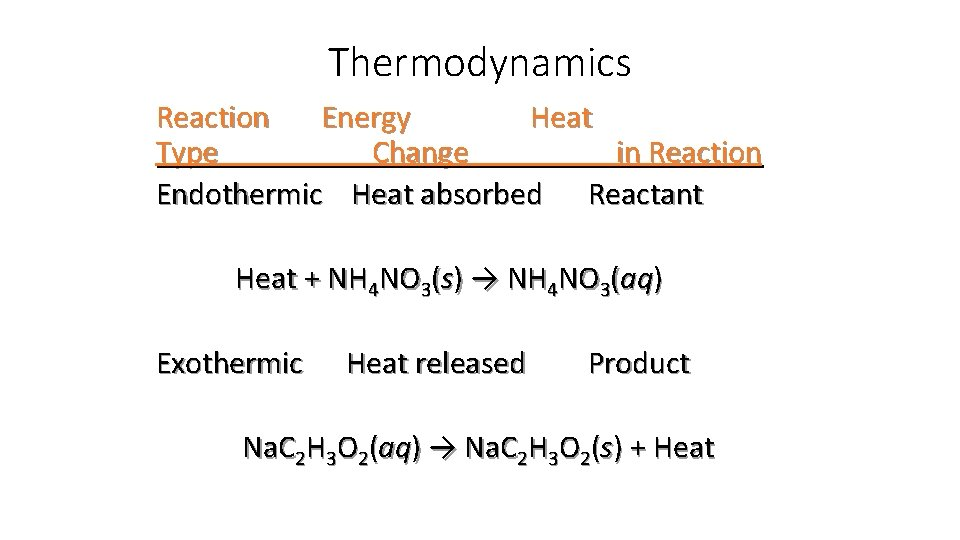Thermodynamics Reaction Energy Heat Type Change in Reaction Endothermic Heat absorbed Reactant Heat + NH 4 NO 3(s) → NH 4 NO 3(aq) Exothermic Heat released Product Na. C 2 H 3 O 2(aq) → Na. C 2 H 3 O 2(s) + Heatspontaneous nonspontaneous The spontaneity of a reaction does not tell us how fast a reaction occurs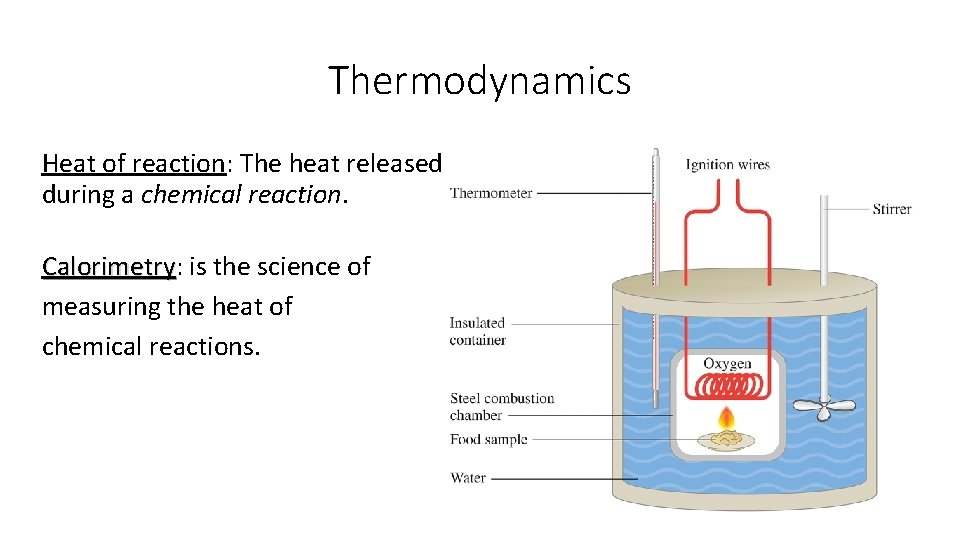Thermodynamics Heat of reaction: The heat released during a chemical reaction. Calorimetry: Calorimetry is the science of measuring the heat of chemical reactions.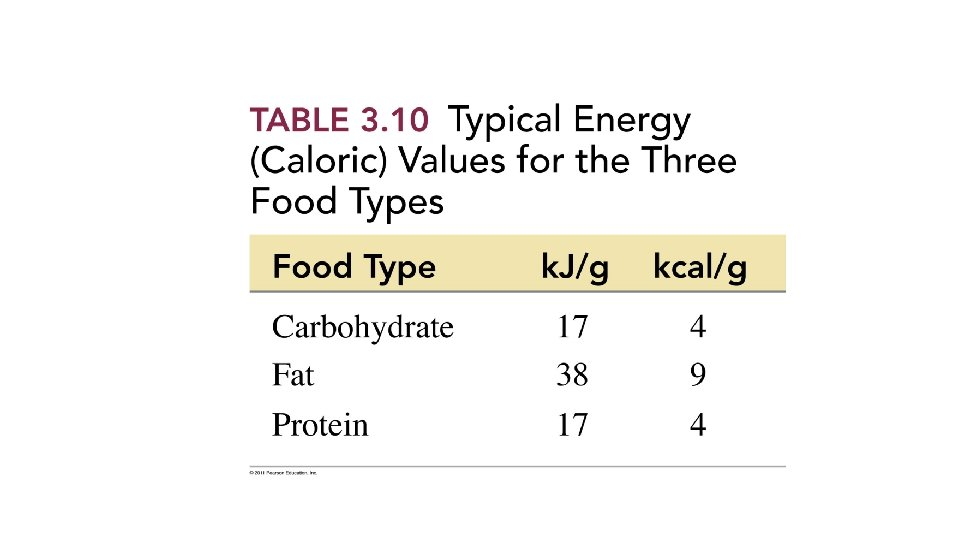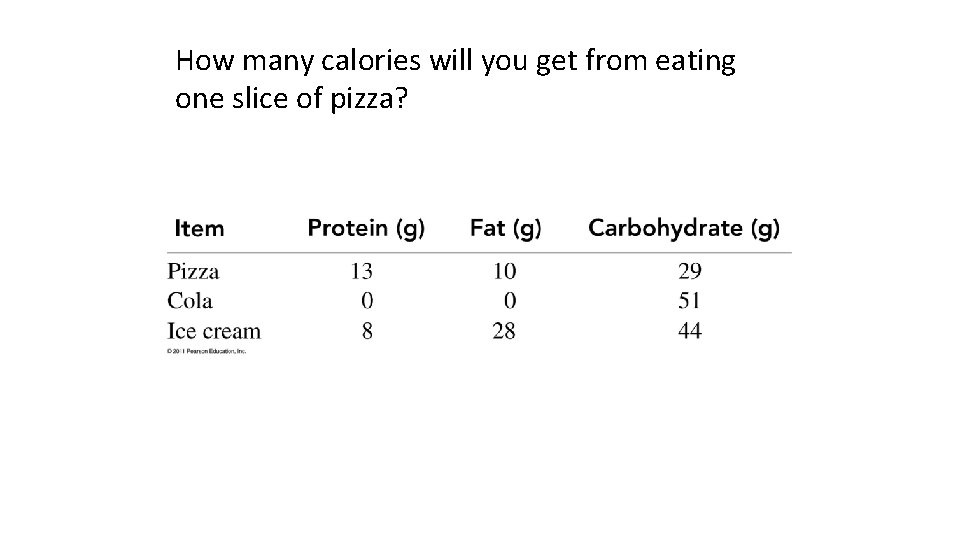How many calories will you get from eating one slice of pizza?Kinetics The rate of reaction is determined by measuring the amount of reactant used up or product formed in a certain period of time. The rate or reaction is affected by • temperature changes. • changes in amount. • the presence of a catalyst.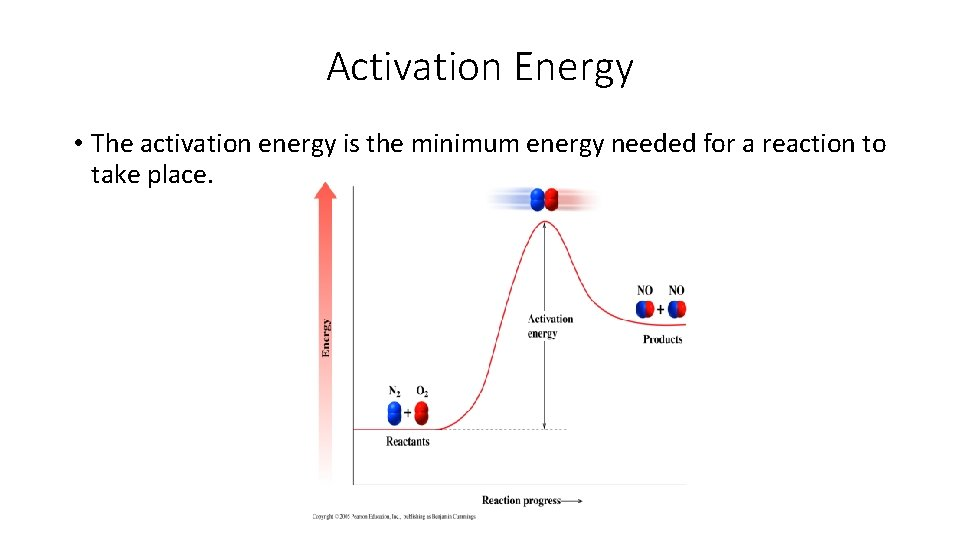Activation Energy • The activation energy is the minimum energy needed for a reaction to take place.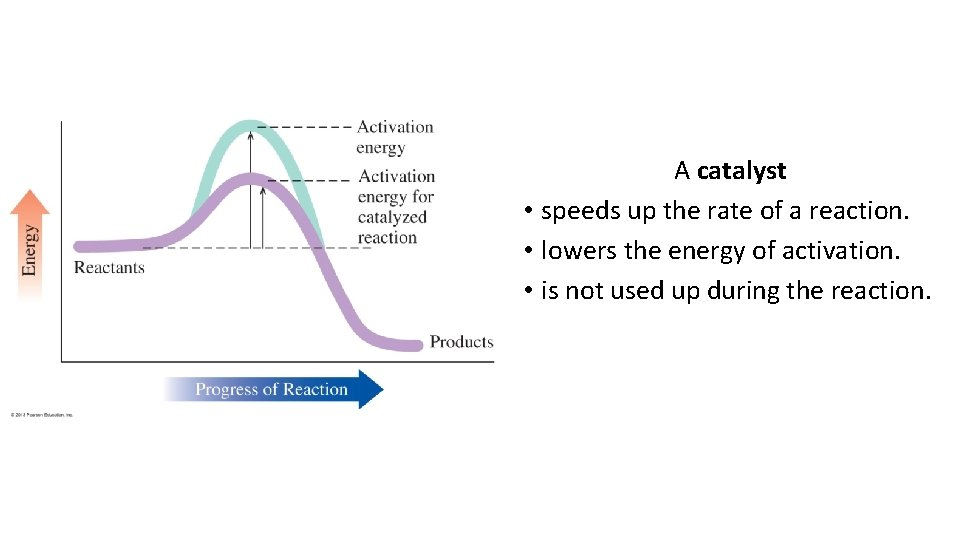A catalyst • speeds up the rate of a reaction. • lowers the energy of activation. • is not used up during the reaction.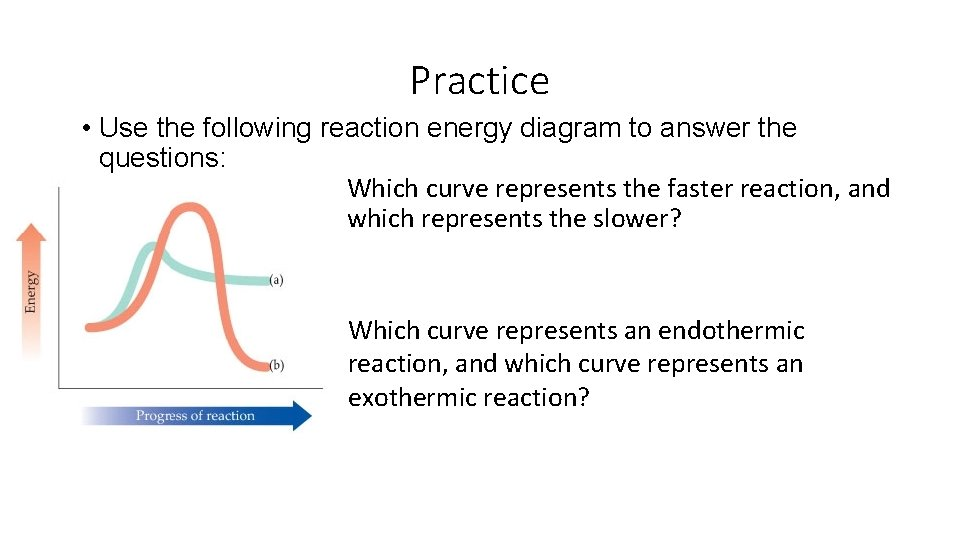Practice • Use the following reaction energy diagram to answer the questions: Which curve represents the faster reaction, and which represents the slower? Which curve represents an endothermic reaction, and which curve represents an exothermic reaction?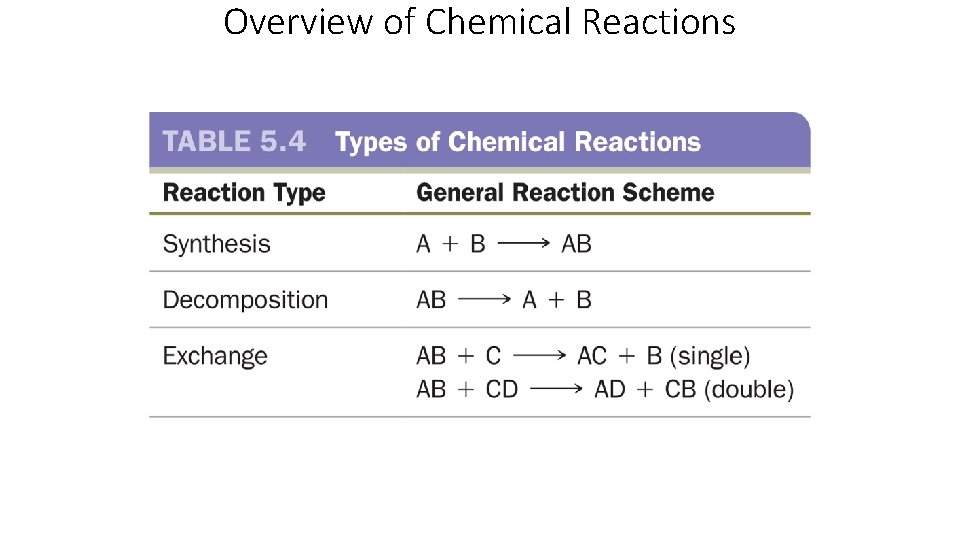Overview of Chemical Reactions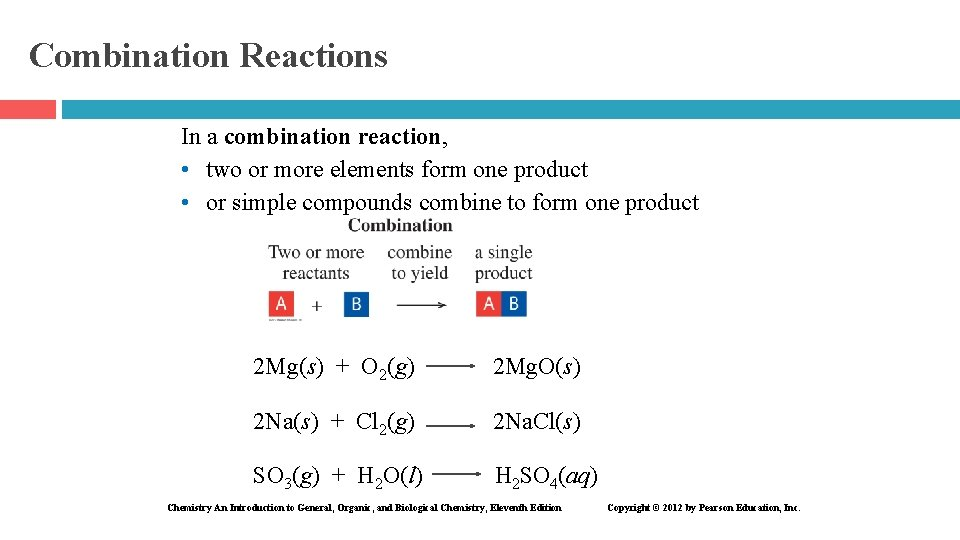Combination Reactions In a combination reaction, • two or more elements form one product • or simple compounds combine to form one product 2 Mg(s) + O 2(g) 2 Mg. O(s) 2 Na(s) + Cl 2(g) 2 Na. Cl(s) SO 3(g) + H 2 O(l) H 2 SO 4(aq) Chemistry An Introduction to General, Organic, and Biological Chemistry, Eleventh Edition Copyright © 2012 by Pearson Education, Inc.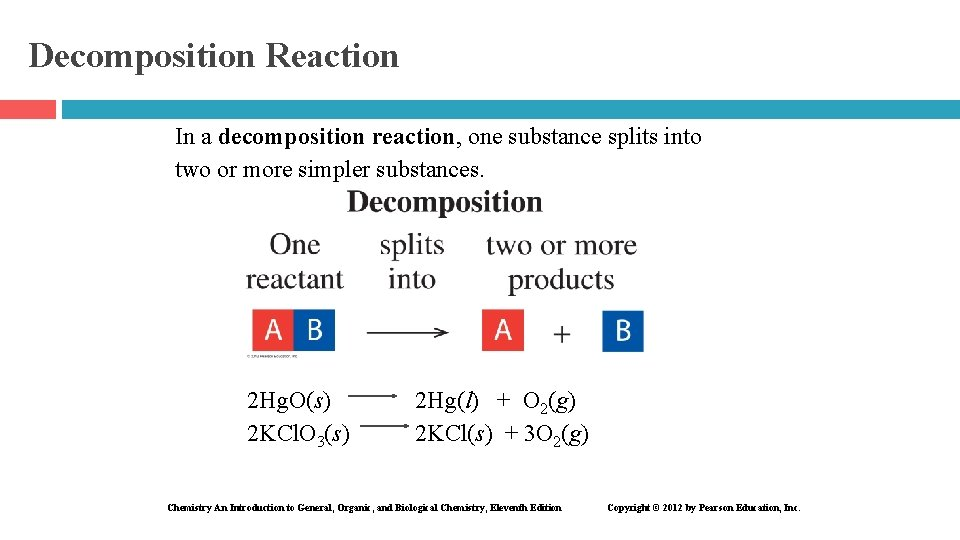Decomposition Reaction In a decomposition reaction, one substance splits into two or more simpler substances. 2 Hg. O(s) 2 KCl. O 3(s) 2 Hg(l) + O 2(g) 2 KCl(s) + 3 O 2(g) Chemistry An Introduction to General, Organic, and Biological Chemistry, Eleventh Edition Copyright © 2012 by Pearson Education, Inc.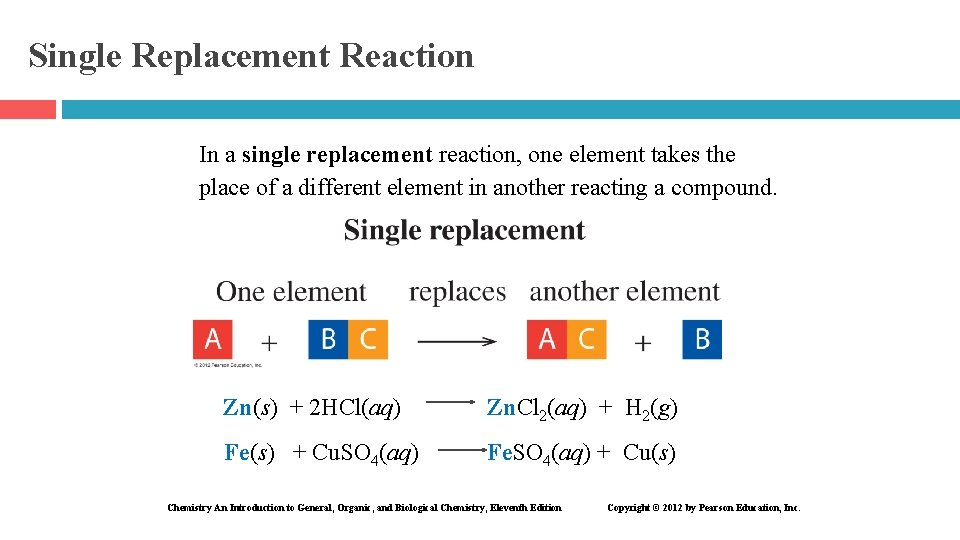Single Replacement Reaction In a single replacement reaction, one element takes the place of a different element in another reacting a compound. Zn(s) + 2 HCl(aq) Zn. Cl 2(aq) + H 2(g) Fe(s) + Cu. SO 4(aq) Fe. SO 4(aq) + Cu(s) Chemistry An Introduction to General, Organic, and Biological Chemistry, Eleventh Edition Copyright © 2012 by Pearson Education, Inc.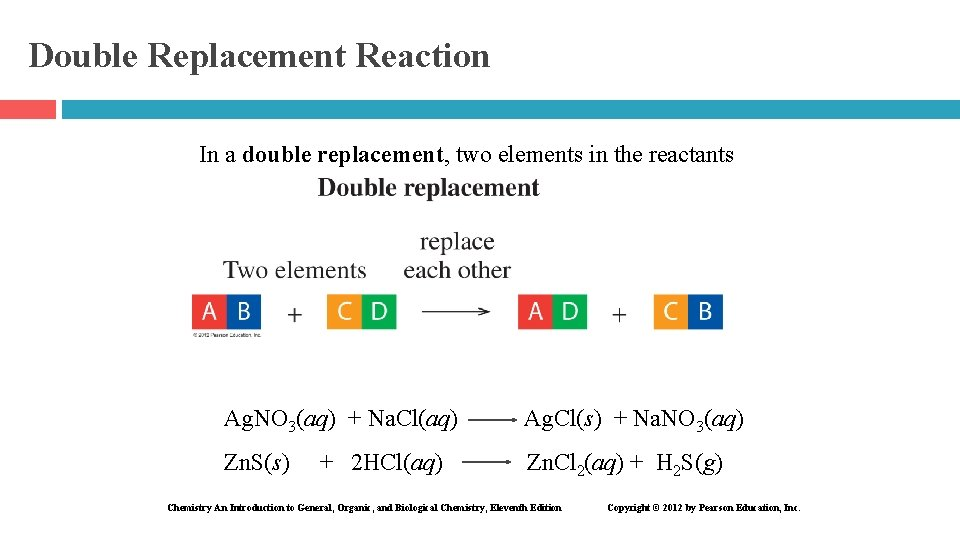Double Replacement Reaction In a double replacement, two elements in the reactants exchange places. Ag. NO 3(aq) + Na. Cl(aq) Ag. Cl(s) + Na. NO 3(aq) Zn. S(s) Zn. Cl 2(aq) + H 2 S(g) + 2 HCl(aq) Chemistry An Introduction to General, Organic, and Biological Chemistry, Eleventh Edition Copyright © 2012 by Pearson Education, Inc.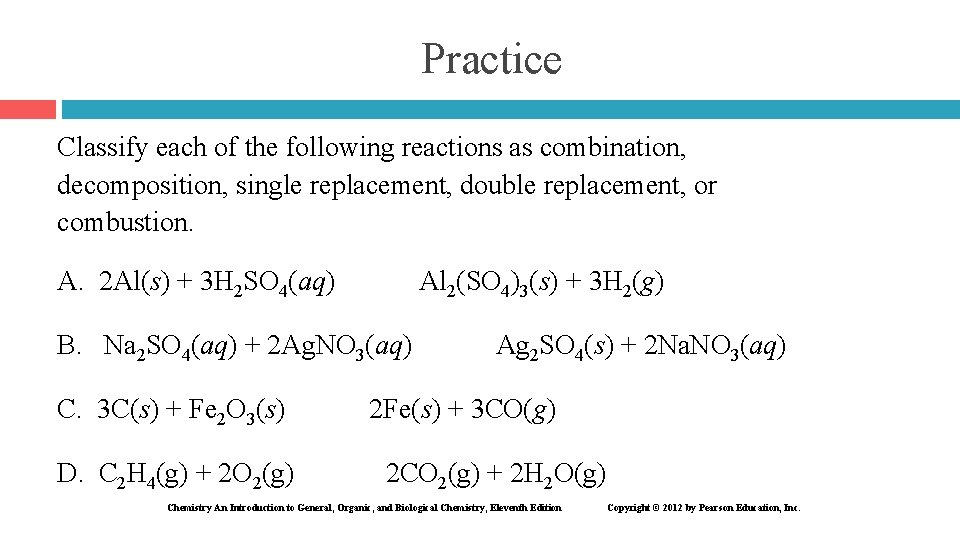Practice Classify each of the following reactions as combination, decomposition, single replacement, double replacement, or combustion. A. 2 Al(s) + 3 H 2 SO 4(aq) Al 2(SO 4)3(s) + 3 H 2(g) B. Na 2 SO 4(aq) + 2 Ag. NO 3(aq) C. 3 C(s) + Fe 2 O 3(s) D. C 2 H 4(g) + 2 O 2(g) Ag 2 SO 4(s) + 2 Na. NO 3(aq) 2 Fe(s) + 3 CO(g) 2 CO 2(g) + 2 H 2 O(g) Chemistry An Introduction to General, Organic, and Biological Chemistry, Eleventh Edition Copyright © 2012 by Pearson Education, Inc.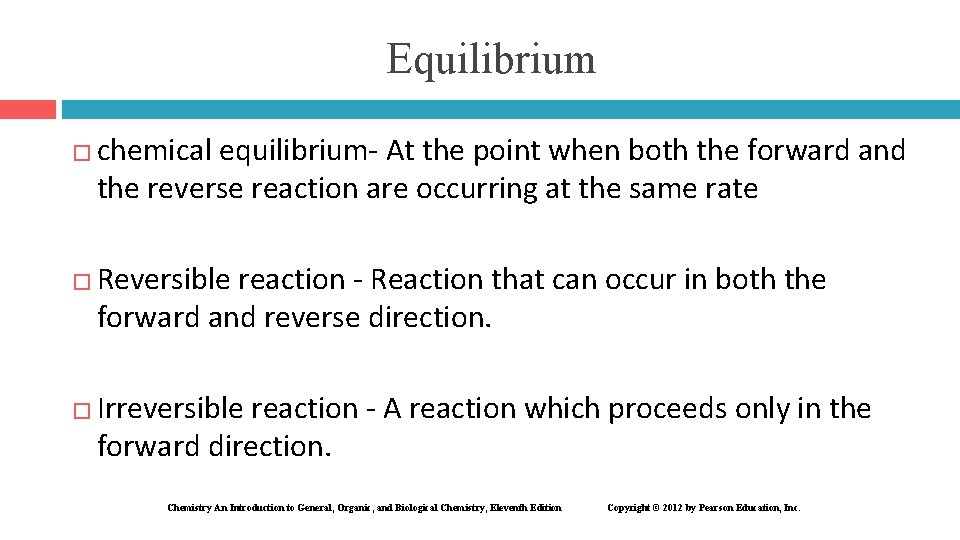Equilibrium � � � chemical equilibrium- At the point when both the forward and the reverse reaction are occurring at the same rate Reversible reaction - Reaction that can occur in both the forward and reverse direction. Irreversible reaction - A reaction which proceeds only in the forward direction. Chemistry An Introduction to General, Organic, and Biological Chemistry, Eleventh Edition Copyright © 2012 by Pearson Education, Inc.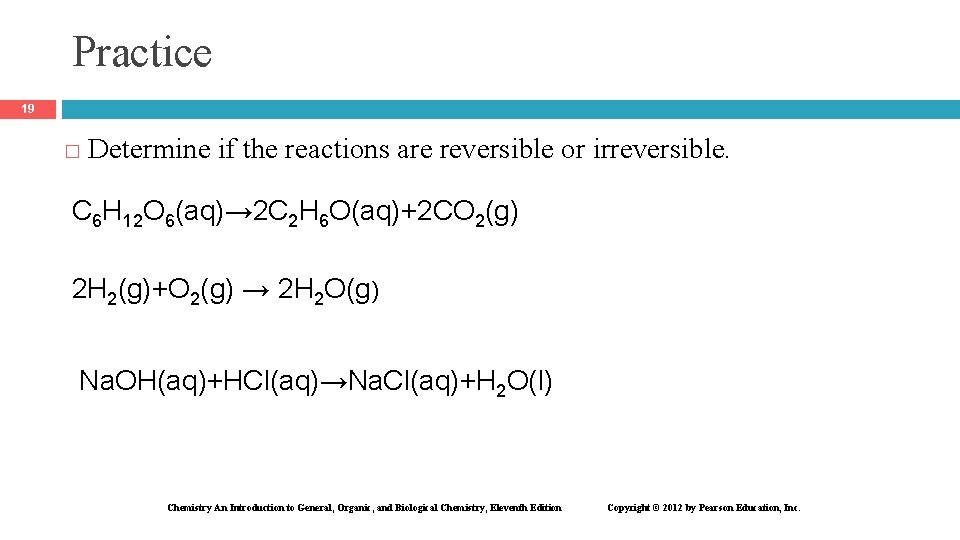Practice 19 � Determine if the reactions are reversible or irreversible. C 6 H 12 O 6(aq)→ 2 C 2 H 6 O(aq)+2 CO 2(g) 2 H 2(g)+O 2(g) → 2 H 2 O(g) Na. OH(aq)+HCl(aq)→Na. Cl(aq)+H 2 O(l) Chemistry An Introduction to General, Organic, and Biological Chemistry, Eleventh Edition Copyright © 2012 by Pearson Education, Inc.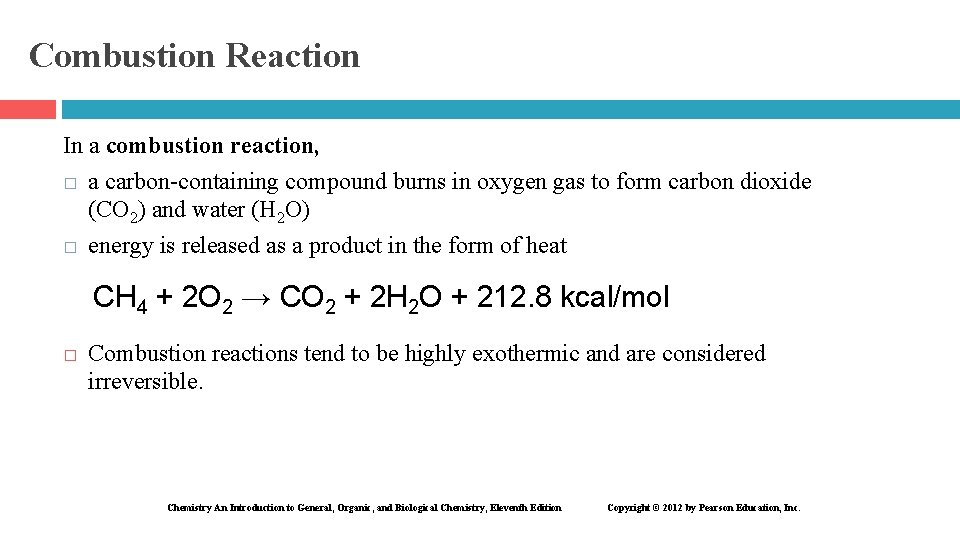Combustion Reaction In a combustion reaction, � a carbon-containing compound burns in oxygen gas to form carbon dioxide (CO 2) and water (H 2 O) � energy is released as a product in the form of heat CH 4 + 2 O 2 → CO 2 + 2 H 2 O + 212. 8 kcal/mol � Combustion reactions tend to be highly exothermic and are considered irreversible. Chemistry An Introduction to General, Organic, and Biological Chemistry, Eleventh Edition Copyright © 2012 by Pearson Education, Inc.Oxidation−Reduction In an oxidation–reduction reaction, electrons are transferred from one substance to another. Chemistry An Introduction to General, Organic, and Biological Chemistry, Eleventh Edition Copyright © 2012 by Pearson Education, Inc.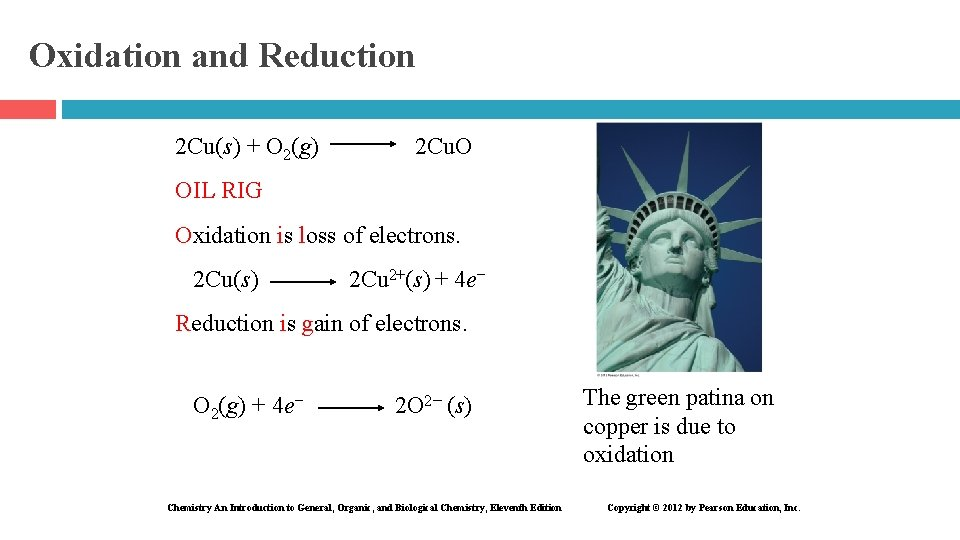Oxidation and Reduction 2 Cu(s) + O 2(g) 2 Cu. O OIL RIG Oxidation is loss of electrons. 2 Cu(s) 2 Cu 2+(s) + 4 e− Reduction is gain of electrons. O 2(g) + 4 e− 2 O 2− (s) Chemistry An Introduction to General, Organic, and Biological Chemistry, Eleventh Edition The green patina on copper is due to oxidation Copyright © 2012 by Pearson Education, Inc.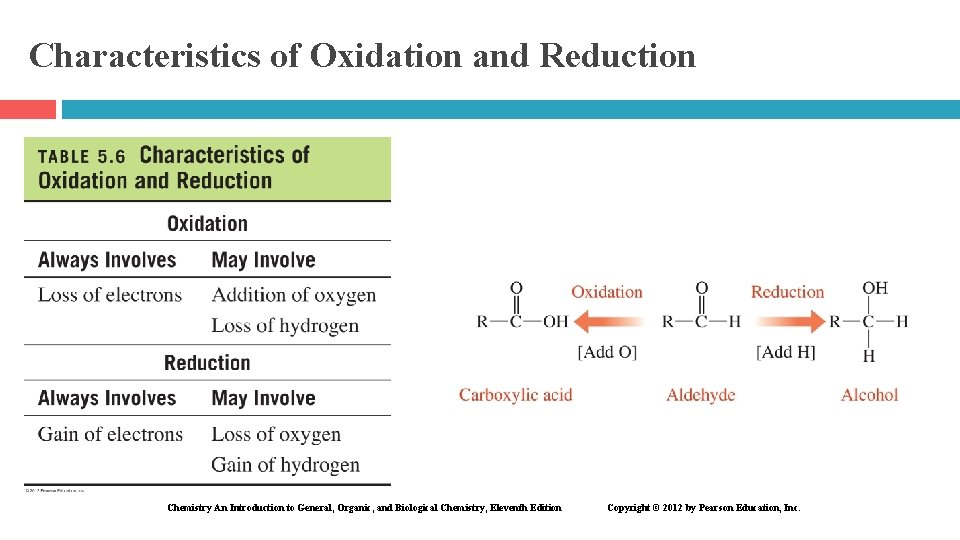Characteristics of Oxidation and Reduction Chemistry An Introduction to General, Organic, and Biological Chemistry, Eleventh Edition Copyright © 2012 by Pearson Education, Inc.Reducing agent: � Loses one or more electrons � Causes reduction � Undergoes oxidation � Becomes more positive (or less negative) Oxidizing agent: � Gains one or more electrons � Causes oxidation � Undergoes reduction � Becomes more negative (or less positive)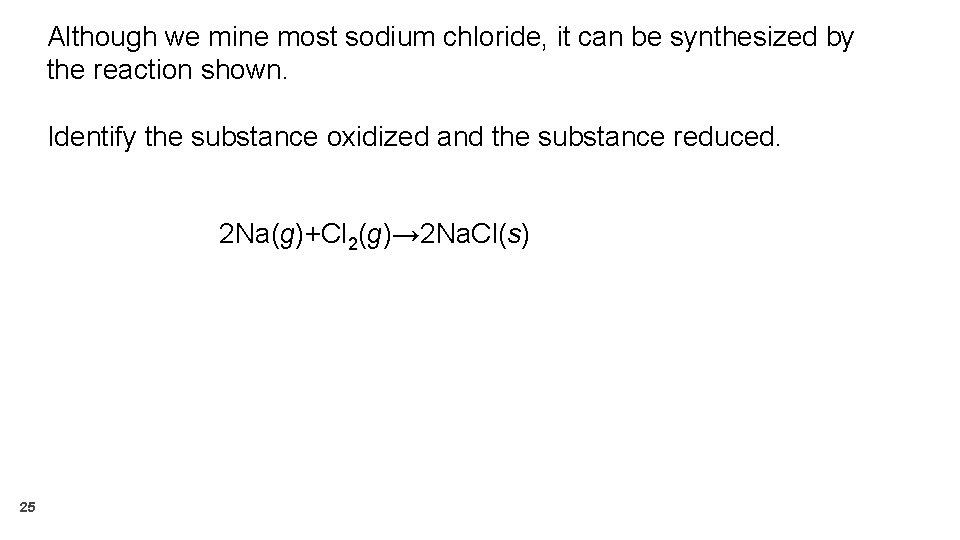Although we mine most sodium chloride, it can be synthesized by the reaction shown. Identify the substance oxidized and the substance reduced. 2 Na(g)+Cl 2(g)→ 2 Na. Cl(s) 25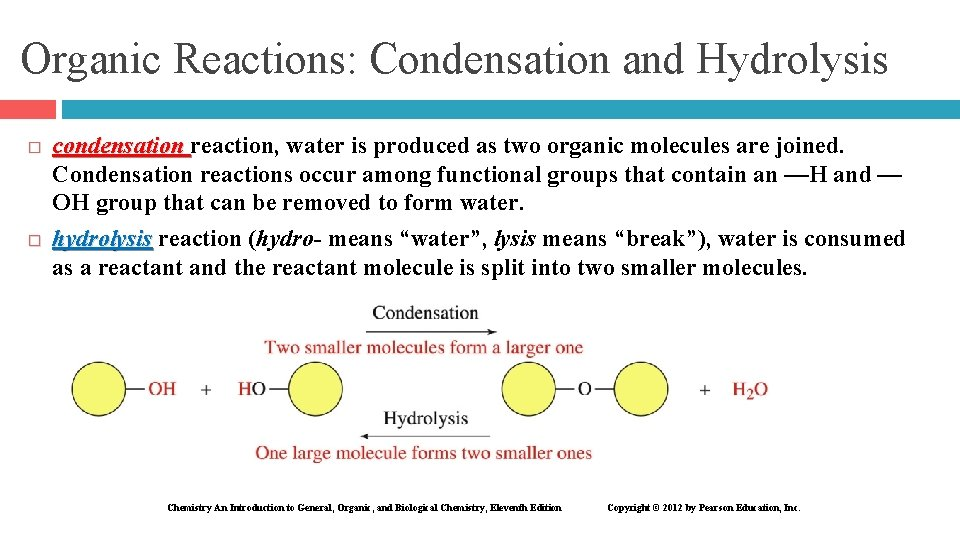Organic Reactions: Condensation and Hydrolysis � � condensation reaction, water is produced as two organic molecules are joined. Condensation reactions occur among functional groups that contain an —H and — OH group that can be removed to form water. hydrolysis reaction (hydro- means “water”, lysis means “break”), water is consumed as a reactant and the reactant molecule is split into two smaller molecules. Chemistry An Introduction to General, Organic, and Biological Chemistry, Eleventh Edition Copyright © 2012 by Pearson Education, Inc.condensationhydrolysis 28Identify the main organic reaction shown as either condensation, hydrolysis, oxidation, hydration, or reduction: 29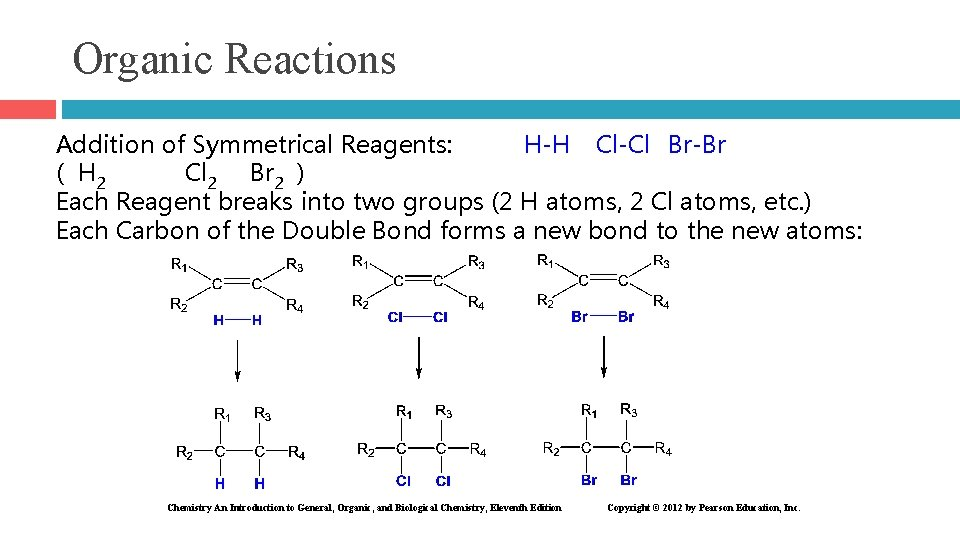Organic Reactions Addition of Symmetrical Reagents: H-H Cl-Cl Br-Br ( H 2 Cl 2 Br 2 ) Each Reagent breaks into two groups (2 H atoms, 2 Cl atoms, etc. ) Each Carbon of the Double Bond forms a new bond to the new atoms: Chemistry An Introduction to General, Organic, and Biological Chemistry, Eleventh Edition Copyright © 2012 by Pearson Education, Inc.Organic Reactions Addition of Unsymmetrical Reagents: H-Cl H-Br H-OH HOCH 3 (HCl HBr H 2 O HOCH 3) Each Reagent breaks into a H atom and the rest of the molecule Each Carbon of the Double Bond forms a new bond to the new groups: Chemistry An Introduction to General, Organic, and Biological Chemistry, Eleventh Edition Copyright © 2012 by Pearson Education, Inc.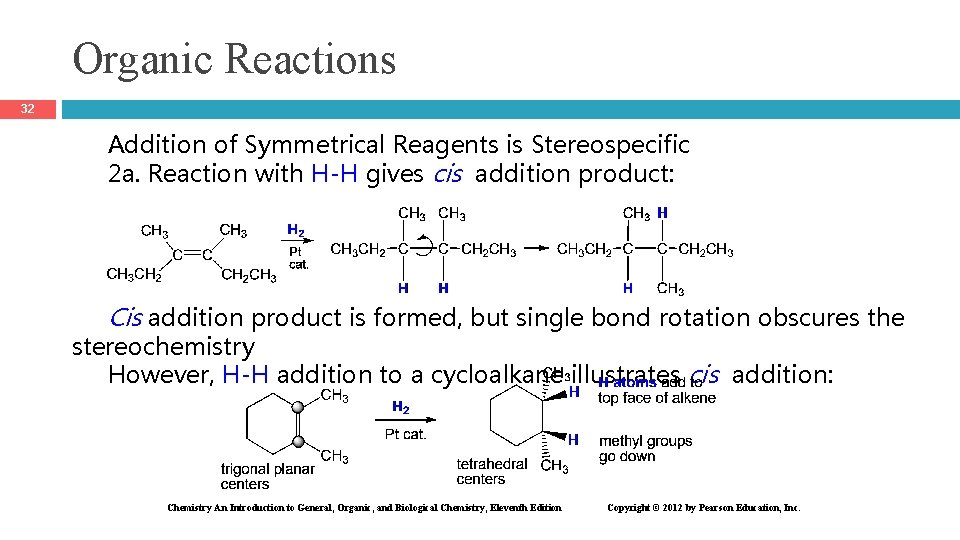Organic Reactions 32 Addition of Symmetrical Reagents is Stereospecific 2 a. Reaction with H-H gives cis addition product: Cis addition product is formed, but single bond rotation obscures the stereochemistry However, H-H addition to a cycloalkane illustrates cis addition: Chemistry An Introduction to General, Organic, and Biological Chemistry, Eleventh Edition Copyright © 2012 by Pearson Education, Inc.Organic Reactions 33 � Addition of Unsymmetrical Reagents is Regiospecific Chemistry An Introduction to General, Organic, and Biological Chemistry, Eleventh Edition Copyright © 2012 by Pearson Education, Inc.Organic Reactions 34 � The same regiochemistry is observed for ALL unsymmetrical reagents: Chemistry An Introduction to General, Organic, and Biological Chemistry, Eleventh Edition Copyright © 2012 by Pearson Education, Inc.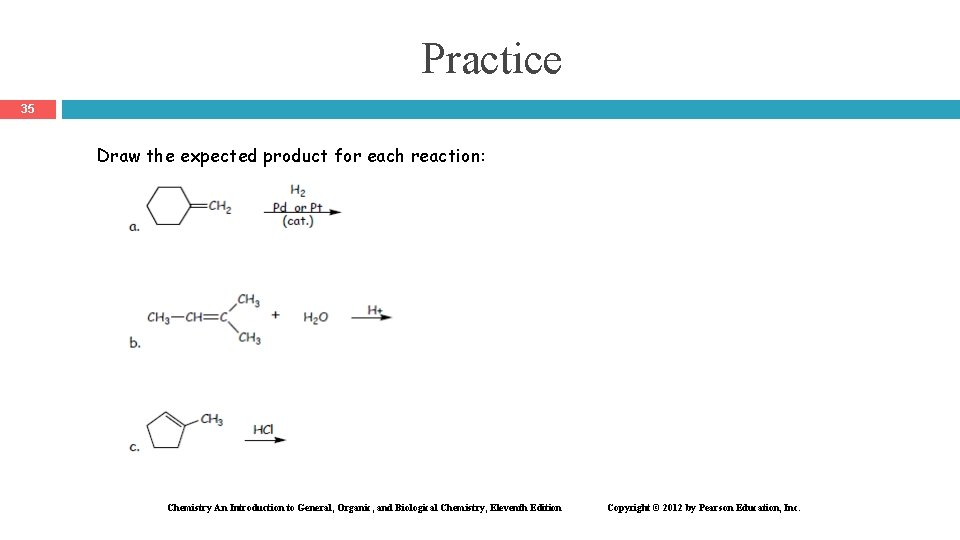Practice 35 Draw the expected product for each reaction: Chemistry An Introduction to General, Organic, and Biological Chemistry, Eleventh Edition Copyright © 2012 by Pearson Education, Inc.